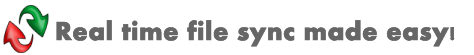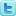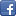Can I use variables in a task?

Beyond Sync supports pre-defined variables, user defined variables and environment variables.

(1) Pre-defined variables

 Variable Name Description %DATE% Current date, for example: 20101209 %TIME% Current time, for example: 121023 %DATETIME% Current date time, for example: 20101209121023 %YEAR2% Two digits year. For example: 07, 08 %YEAR4% Four digits year. For example: 2007, 2008 %MONTH% Use one digit for month when it less than 10 %MONTH2% Always use two digits for month. For example: 01, 02, 10, 12 %DAY% Use one digit for day when it less than 10 %DAY2% Always use two digits for day. For example: 01, 02, 10 %HOUR% Use one digit for hour when it less than 10 %HOUR2% Always use two digits. For example: 01, 02 %MINUTE% Use one digit for minute when it less then 10 %MINUTE2% Always use two digits for minute. For example: 01, 02, 10 %SECOND% Use one digit for second when it less than 10 %SECOND2% Always use two digits for second. For example: 01, 02, 10

(2) User defined variables

(3) Environment variables

You can also specify any environment variable in Task Folder or Log File paths. For example:

Source Folder: c:\sourceTwitter |Facebook